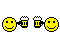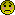###Author Topic: 2D Torus knot drawing  (Read 2641 times)

0 Members and 1 Guest are viewing this topic.

####KrazyK##### 2D Torus knot drawing
« on: April 03, 2013 »
Here's a snippet of code that I used in my D-Bug 178 remake for drawing the torus shapes.
If someone can adapt this to produce a 3D torus knot then please show me as it's been driving me nuts!

Code: [Select]
`;- Simple 2D Torus drawing routine;-Play around with the values below and try your own design - do your own boundary checking!P.f=8Q.f=3values=256*2 ;-number of points in the torust.f=0stp.f=(#PI*2)/valuesscrw=640scrh=480InitSprite()OpenWindow(1,0,0,scrw,scrh,"",#PB_Window_ScreenCentered|#PB_Window_WindowCentered)OpenWindowedScreen(WindowID(1),0,0,scrw,scrh,0,0,0)Repeat t.f+stp R.f=0.7*(2+Sin(P*t)) xpos=R*(Cos(Q*t)*80) ypos=R*(Cos(P*t)) zpos=R*Sin(Q*t)*80 StartDrawing(ScreenOutput()) Box(xpos+300,ypos+250+zpos,1,1,RGB(229,194,4)) StopDrawing() FlipBuffers() If t>#PI*2:ClearScreen(0):t=0:EndIfUntil GetAsyncKeyState_(#VK_ESCAPE)`

####relsoft

•• Posts: 3303
• Karma: 47##### Re: 2D Torus knot drawing
« Reply #1 on: April 06, 2013 »
You could use a frenet frame to extrude points from the center.

Code: [Select]
`'PQTorus Knot Generator'Special thanks to both these guys for the docs they made:'BlackPawn = for the Algo of the knot and the Frenet square approximation'http://www.blackpawn.com/texts/pqtorus/default.html'Jari Komppa aka Sol / Trauma(I used his wave-algo an a lot of stuff)'Relsoft 2k9'Rel.Betterwebber.comconst SCR_WIDTH = 640                   'Width of the screen const SCR_HEIGHT = 480                  'height of the screen const BPP = 32                       '32 bits per pixel format const SCR_MIDX = SCR_WIDTH  \ 2const SCR_MIDY = SCR_HEIGHT \ 2const PI = 3.141593                     'PI for rotation const TWOPI = (2 * PI)const LENS = 256                            'zconst TOR_NUM_RINGS = 360const TOR_NUM_BANDS = 64const TOR_RING_RAD  = 2.0const TOR_BAND_RAD  = 0.5Type vector3d x as single y as single z as singleend typedeclare sub vector_cross (v1 as vector3d, v2 as vector3d, vout as vector3d)declare function vector_magnitude(v as vector3d) as singledeclare sub vector_normalize (v as vector3d)declare sub vector_add (v1 as vector3d, v2 as vector3d, vout as vector3d)declare sub vector_sub (v1 as vector3d, v2 as vector3d, vout as vector3d)declare sub  DrawPQTorus (byval Rings as integer, byval Bands as integer,_                        byval RINGRADIUS as single, byval BandRadius as single,_                        fold_off as single, foldnum as single, fold_scale as single)'/******************************************************************************************'/******************************************************************************************dim fold_off as singledim fold_num as singledim fold_scale as singlefold_off = 0.02fold_scale = 0.05fold_num = 16screenres SCR_WIDTH,SCR_HEIGHT,BPP,2DrawPQTorus (TOR_NUM_RINGS, TOR_NUM_BANDS, TOR_RING_RAD, TOR_BAND_RAD,fold_off, fold_num, fold_scale)sleepend'/******************************************************************************************'/******************************************************************************************sub  DrawPQTorus (byval Rings as integer, byval Bands as integer,_                  byval RINGRADIUS as single, byval BandRadius as single,_                  fold_off as single, foldnum as single, fold_scale as single)    dim x as single, y as single, z as single dim oq as single, op as single dim max_radius  as single oq = 4 op = 3 max_radius = 2.0    for i as integer = 0 to rings - 1      dim current_point as vector3d        dim next_point as vector3d        dim T as vector3d        dim B as vector3d        dim N as vector3d        dim mag as single        dim p as single        dim q as single        dim r as single        'center point        p = op * i * TWOPI / rings   'p and q        q = oq * i * TWOPI / rings   'interpolate        r = (.5 * (2 + sin(q))) * max_radius   'radius of each ring        current_point.x = r * cos(p)   'rotate point        current_point.y = r * cos(q)        current_point.z = r * sin(p)        'next point for Frenet square        p = op * (i + 1) * TWOPI / rings        q = oq * (i + 1) * TWOPI / rings        r = (.5 * (2 + sin(q))) * max_radius        next_point.x = r * cos(p)        next_point.y = r * cos(q)        next_point.z = r * sin(p)        'T  = P' - P        vector_sub next_point, current_point, T        'N = P' + P        vector_add next_point, current_point, N        'B = T x N        vector_cross T, N, B        'N = B x T        vector_cross B, T, N        'Normalize vectors or else it won't work        vector_normalize B        vector_normalize N        for j as integer = 0 to bands - 1            dim new_point_x as single        dim new_point_y as single                'rotate around the current point using normal rotation makes bands            new_point_x = sin(j * TWOPI / bands) * TOR_BAND_RAD            new_point_y = cos(j * TWOPI / bands) * TOR_BAND_RAD                        'waves(2d flower algo)            new_point_x = new_point_x * ((sin(fold_off + foldnum * i * TWOPI / rings) * fold_scale) + 1)            new_point_y = new_point_y * ((cos(fold_off + foldnum * i * TWOPI / rings) * fold_scale) + 1) 'this is the coords of our point along the curve            x = N.x * new_point_x + B.x * new_point_y + current_point.x            y = N.y * new_point_x + B.y * new_point_y + current_point.y            z = N.z * new_point_x + B.z * new_point_y + current_point.z                        'project            dim as single scaler = 40  'this makes the model bigger            dim as single distance = (LENS - z*scaler)            dim as single xr,yr            if distance > 0 then                xr = SCR_MIDX + (LENS * x*scaler / distance)                yr = SCR_MIDY - (LENS * y*scaler / distance)                pset(xr,yr),rgb(255,255,25)            end if sleep 1,1        next     next end subsub vector_cross (v1 as vector3d, v2 as vector3d, vout as vector3d)    vout.x = (v1.y * v2.z) - (v2.y * v1.z)    vout.y = (v1.z * v2.x) - (v2.z * v1.x)    vout.z = (v1.x * v2.y) - (v2.x * v1.y)end subfunction vector_magnitude(v as vector3d) as single    dim mag as single    mag = sqr(v.x * v.x + v.y * v.y + v.z * v.z)    if mag = 0 then mag = 1    vector_magnitude = magend functionsub vector_normalize (v as vector3d)    dim mag as single    mag = vector_magnitude(v)    v.x = v.x / mag    v.y = v.y / mag    v.z = v.z / magend subsub vector_add (v1 as vector3d, v2 as vector3d, vout as vector3d)    vout.x = v1.x + v2.x    vout.y = v1.y + v2.y    vout.z = v1.z + v2.zend subsub vector_sub (v1 as vector3d, v2 as vector3d, vout as vector3d)    vout.x = v1.x - v2.x    vout.y = v1.y - v2.y    vout.z = v1.z - v2.zend sub`

####Raizor

• Founder Member
• Pentium
••• Posts: 1150
• Karma: 174##### Re: 2D Torus knot drawing
« Reply #2 on: April 06, 2013 »
This is worth checking out: http://www.blackpawn.com/texts/pqtorus/

####C3lt1c##### Re: 2D Torus knot drawing
« Reply #3 on: April 06, 2013 »
Awesome stuff bro's!
Thx for sharing it !Cheers C3lt1c
OLD SCHOOL ROCKZZZ

####ninogenio

• Pentium
•• Posts: 1666
• Karma: 133##### Re: 2D Torus knot drawing
« Reply #4 on: April 07, 2013 »
excellent stuff guys this is something ive been wanting too try for a while,

looks like working between Raizors link and rels code, some really cool stuff can be done.

####KrazyK##### Re: 2D Torus knot drawing
« Reply #5 on: April 07, 2013 »
Please, someone convert relsoft's code to PB.  I'd love to try it out !####relsoft

•